Courses

# Signal To Noise Ratio Electronics and Communication Engineering (ECE) Notes | EduRev

## Communication Theory

Created by: Machine Experts

## Electronics and Communication Engineering (ECE) : Signal To Noise Ratio Electronics and Communication Engineering (ECE) Notes | EduRev

The document Signal To Noise Ratio Electronics and Communication Engineering (ECE) Notes | EduRev is a part of the Electronics and Communication Engineering (ECE) Course Communication Theory.
All you need of Electronics and Communication Engineering (ECE) at this link: Electronics and Communication Engineering (ECE)

SIGNAL-TO-NOISE RATIO:

Signal-to-noise ratio (often abbreviated SNR or S/N) is a measure used in science and engineering to quantify how much a signal has been corrupted by noise. It is defined as the ratio of signal power to the noise power corrupting the signal. A ratio higher than 1:1 indicates more signal than noise. While SNR is commonly quoted for electrical signals, it can be applied to any form of signal (such as isotope levels in an ice core or biochemical signaling between cells).

In less technical terms, signal-to-noise ratio compares the level of a desired signal (such as music) to the level of background noise. The higher the ratio, the less obtrusive the background noise is

. "Signal-to-noise ratio" is sometimes used informally to refer to the ratio of useful information to false or irrelevant data in a conversation or exchange. For example, in online discussion forums and other online communities, off-topic posts and spam are regarded as "noise" that interferes with the "signal" of appropriate discussion.

Signal-to-noise ratio is defined as the power ratio between a signal (meaningful information) and the background noise (unwanted signal):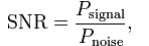where P is average power. Both signal and noise power must be measured at the same or equivalent points in a system, and within the same system bandwidth. If the signal and the noise are measured across the same impedance, then the SNR can be obtained by calculating the square of the amplitude ratio: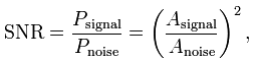where A is root mean square (RMS) amplitude (for example, RMS voltage). Because many signals have a very wide dynamic range, SNRs are often expressed using the logarithmicdecibel scale. In decibels, the SNR is defined as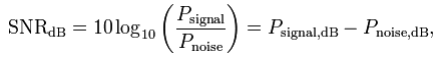which may equivalently be written using amplitude ratios as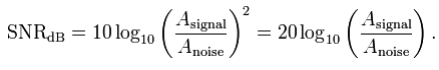The concepts of signal-to-noise ratio and dynamic range are closely related. Dynamic range measures the ratio between the strongest un-distorted signal on a channel and the minimum discernable signal, which for most purposes is the noise level. SNR measures the ratio between an arbitrary signal level (not necessarily the most powerful signal possible) and noise. Measuring signal-to-noise ratios requires the selection of a representative or reference signal. In audio engineering, the reference signal is usually a sine wave at a standardized nominal or alignment level, such as 1 kHz at +4 dBu (1.228 VRMS).

SNR is usually taken to indicate an average signal-to-noise ratio, as it is possible that (near) instantaneous signal-to-noise ratios will be considerably different. The concept can be understood as normalizing the noise level to 1 (0 dB) and measuring how far the signal 'stands out'.

Mutual information:

In probability theory and information theory, the mutual information (sometimes known by the archaic term trans information) of two random variables is a quantity that measures the mutual dependence of the two variables. The most common unit of measurement of mutual information is the bit, when logarithms to the base 2 are used.

Definition of mutual information:

Formally, the mutual information of two discrete random variables X and Y can be defined as: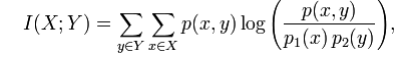where p(x,y) is the joint probability distribution function of X and Y, and p1(x) and p2(y) are the marginal probability distribution functions of X and Y respectively. In the case of a continuous function, summation is matched with a definite double integral: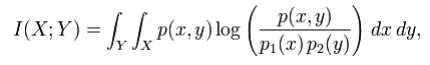where p(x,y) is now the joint probability density function of X and Y, and p1(x) and p2(y) are the marginal probability density functions of X and Y respectively.

These definitions are ambiguous because the base of the log function is not specified. To disambiguate, the function I could be parameterized as I(X,Y,b) where b is the base. Alternatively, since the most common unit of measurement of mutual information is the bit, a base of 2 could be specified.

Intuitively, mutual information measures the information that X and Y share: it measures how much knowing one of these variables reduces our uncertainty about the other. For example, if X and Y are independent, then knowing X does not give any information about Y and vice versa, so their mutual information is zero. At the other extreme, if X and Y are identical then all information conveyed by X is shared with Y: knowing X determines the value of Y and vice versa. As a result, in the case of identity the mutual information is the same as the uncertainty contained in Y (or X) alone, namely the entropy of Y (or X: clearly if X and Y are identical they have equal entropy).

Mutual information quantifies the dependence between the joint distribution of X and Y and what the joint distribution would be if X and Y were independent. Mutual information is a measure of dependence in the following sense: I(X; Y) = 0 if and only if X and Y are independent random variables. This is easy to see in one direction: if X and Y are independent, then p(x, y) = p(x) p(y), and therefore: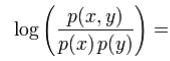log 1 = 0

Moreover, mutual information is nonnegative (i.e. I(X;Y) ≥ 0; see below) and symmetric (i.e. I(X;Y) = I(Y;X)).

Offer running on EduRev: Apply code STAYHOME200 to get INR 200 off on our premium plan EduRev Infinity!

,

,

,

,

,

,

,

,

,

,

,

,

,

,

,

,

,

,

,

,

,

;